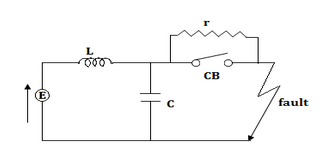# Numerical - Value of 'r' for critical damping in a given CB circuit with L-C damping

Q.  For the following figure shows a CB circuit with L - C introducing a damping circuit. For the critical damping what is the value of r?- Published on 17 Nov 15

a. √(LC)
b. 0.5*√(C/L)
c. 0.5*√(L/C)
d. 2π*√(L/C)